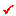Convert the values of the vertical components of the entered loads (loads acting in GCS (-)Z-direction) to masses and enter them as lumped mass data.

Note

This function does not convert self-weight into masses. In order to convert self-weight into masses, "Converting Type of Model Weight to Masses" in Model>Structure Type can be used.

####Mass Direction

Assign applicable directions for the converted masses.

Note
When considering the seismic effect (response spectrum analysis and time history analysis using seismic data), the vertical mass component is usually neglected because the lateral behavior is typically dominant. Thus, it is common to select the X- and Y- mass directions.
However, when considering the floor vibration analysis, it is common to select the Z- mass direction because the vibration mode in the vertical direction is the major mode.

####Load Type for Converting

Select the vertical load types to be converted.

Gravity: Acceleration of gravity

####Load Case/ Factor

Scale Factor: Enter a scale factor to be applied to convert the loads to masses.

Note 1

The general methods of converting the structure and the loads into masses are as follows:

1.Self-weight of the structure

Converting Type of Model Weight to Masses in Model>Structure Type

2. Loads applied to the structure

Note 2

It is common to convert the structure and loads into lumped masses in case of building structures.

Note 3

The converted masses from the structure and the loads can be checked from Query>Mass Summary Table.